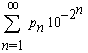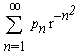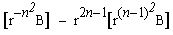# FAQ: Is there a formula for the nth Prime?

### By Chris Caldwell

Is there a formula for producing a specific prime number? Let me give you an example. The formula is given the number 52. In return, the formula produces 239, the fifty-second prime number (I think).

Yes, there are many such formulas--but they are of recreational use only because they are very inefficient. Most are either ways of encoding the list of primes or very clever counting arguments. I will give a couple example below, but for more information start with chapter three "Are There Functions Defining Prime Numbers?" of Ribenboim's text [Ribenboim95pp. 179-212].

### Method One: Encoding Primes

Let pn be the nth prime. In 1952 Sierpinski suggested we define a constant A as follows:

A == 0.02030005000000070...
Then using the floor function [x] (the greatest integer less than or equal to x) we have

pn =Hardy and Wright [HW79p345] give a variant of this: Let r be an integer greater than one and define a constant B as follows:

B =then

pn =This type formula would only be of value if the necessary constant could be found without first finding the primes--this may be possible, but it seems unlikely.

### Method Two: Counting Primes with Wilson's Theorem

First use one of these three methods to define π(x). Willans (1964) used

π(n) = (sum from j=2 to n) sin2(pi*(j-1)!2/j) / sin2(pi/j).

Minác (unpublished, proof in [Ribenboim95, p181]) set

π(n) = (sum from j=2 to n) [ ((j-1)! + 1)/j - [(j-1)!/j] ].

Hardy and Wright set π(1) = 0, π(2) = 1, and then [HW79p414]

π(n) = 1 + (sum from j=3 to n) ( (j-2)! - j[(j-2)!/j] ).

(for all n > 2). Then we have (still using the floor function [x]):

nth prime = 1 + (sum from m=1 to j=2n) [ [ n/(1 + π(m)) ]1/n ]